Want to share your content on R-bloggers? click here if you have a blog, or here if you don't.

### Introduction

This is the 9th post in the series Elegant Data Visualization with
ggplot2
. In the previous post, we learnt how to build bar charts. In this
post, we will learn to:

• build box plots
• modify box
• color
• fill
• alpha
• line size
• line type
• modify outlier
• color
• shape
• size
• alpha

The box plot is a standardized way of displaying the distribution of data. It
is useful for detecting outliers and for comparing distributions and shows the
shape, central tendancy and variability of the data.

## Structure

• the body of the boxplot consists of a “box” (hence, the name), which goes from the first quartile (Q1) to the third quartile (Q3)
• within the box, a vertical line is drawn at the Q2, the median of the data set
• two horizontal lines, called whiskers, extend from the front and back of the box
• the front whisker goes from Q1 to the smallest non-outlier in the data set, and the back whisker goes from Q3 to the largest non-outlier
• if the data set includes one or more outliers, they are plotted separately as points on the chart

### Libraries, Code & Data

We will use the following libraries in this post:

All the data sets used in this post can be found here and code can be downloaded from here.

## Data

We are going to use two different data sets in this post. Both the data sets have the same data but are in
different formats.

```daily_returns <- readr::read_csv('https://raw.githubusercontent.com/rsquaredacademy/datasets/master/tickers.csv')
daily_returns
## # A tibble: 250 x 5
##       AAPL   AMZN      FB    GOOG    MSFT
##
##  1  1.38    24.2   2.12    22.4    1.12
##  2  2.83     3.25 -0.860    5.99   0.767
##  3 -0.0394   9.91  1.45     6.75   0.973
##  4  0.108    3.76 -0.770  -10.7   -0.285
##  5  1.64    19.8   4.75     8.66   0.501
##  6  0.0689   5.33 -0.300   -0.930  0.256
##  7 -0.561   -5.21 -0.630   -7.28  -0.708
##  8  0.551    0.25 -0.460    0.690  0.128
##  9 -0.217  -13.6   0.0300   6.56   0.0786
## 10 -0.108   -4.25  0.460    2.60   0.472
## # ... with 240 more rows```

## Univariate Box Plot

If you are not comparing the distribution of continuous data, you can create
box plot for a single variable. Unlike `plot()`, where we could just use
1 input, in ggplot2, we must specify a value for the X axis and it must be
categorical data. Since we are not comparing distributions, we will use `1`
as the value for the X axis and wrap it inside `factor()` to treat it as a
categorical variable. In the below example, we examine the distribution of
stock returns of Apple.

```ggplot(daily_returns) +
geom_boxplot(aes(x = factor(1), y = AAPL))```## Data

For the rest of the post, we will use the below data set. Instead of 5 columns,
we have two columns. One for the stock names and another for returns.

```tidy_returns <-
col_types = list(col_factor(levels = c('AAPL', 'AMZN', 'FB', 'GOOG', 'MSFT')), col_double()))
tidy_returns
## # A tibble: 1,254 x 2
##    stock returns
##
##  1 AAPL   1.38
##  2 AAPL   2.83
##  3 AAPL  -0.0394
##  4 AAPL   0.108
##  5 AAPL   1.64
##  6 AAPL   0.0689
##  7 AAPL  -0.561
##  8 AAPL   0.551
##  9 AAPL  -0.217
## 10 AAPL  -0.108
## # ... with 1,244 more rows```

## Box Plot

With the above data, let us create a box plot where we compate the distribution
of stock returns of different companies. We map X axis to the column with stock
names and Y axis to the column with stock returns. Note that, the column names
are wrapped inside `aes()`.

```ggplot(tidy_returns) +
geom_boxplot(aes(x = stock, y = returns))```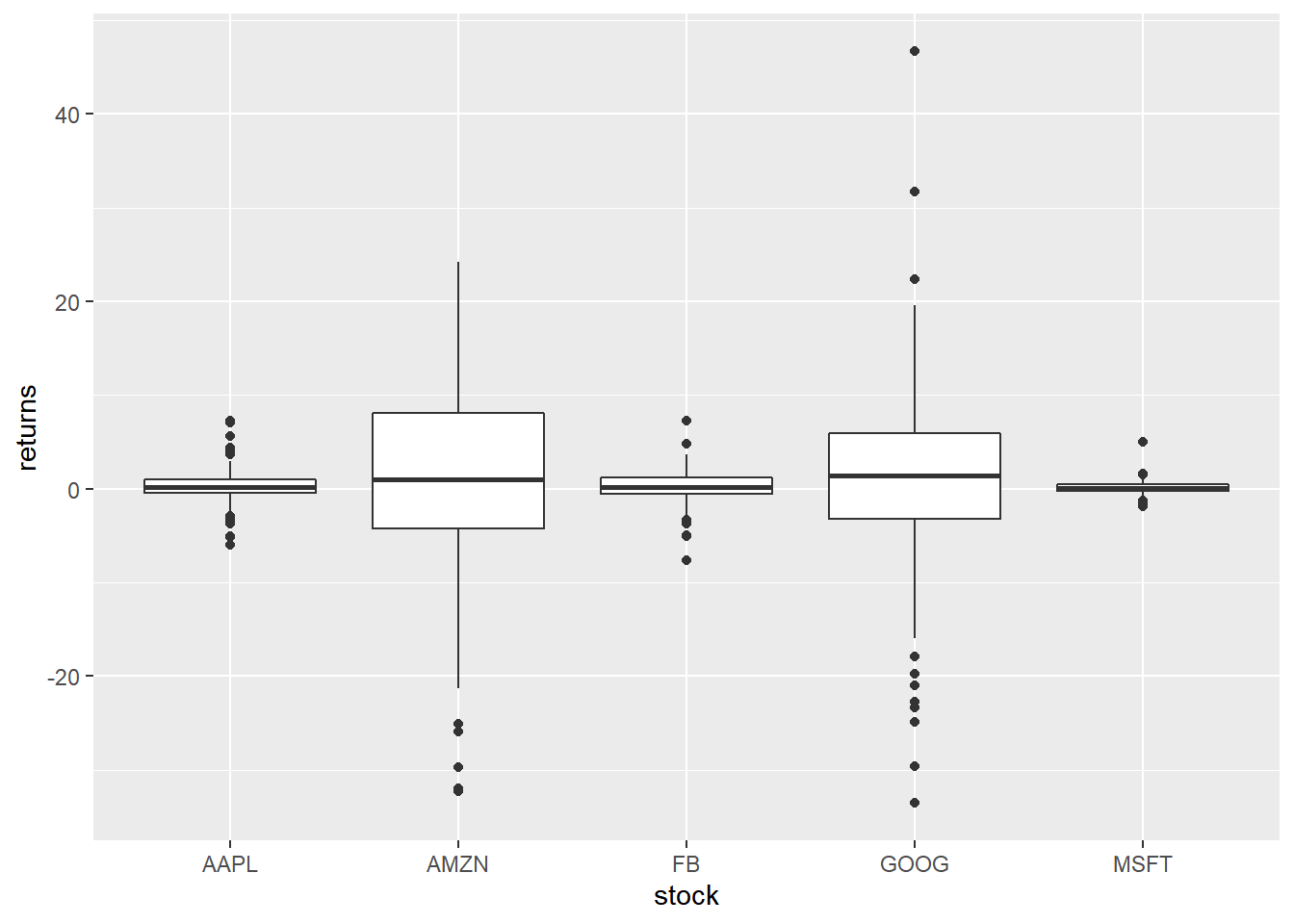To create a horizontal bar plot, we can use `coord_flip()` which will flip the
coordinate axes.

## Horizontal Box Plot

```ggplot(tidy_returns) +
geom_boxplot(aes(x = stock, y = returns)) +
coord_flip()```## Notch

Notches are used to compare medians. You can use the `notch` argument and set
it to `TRUE`.

```ggplot(tidy_returns) +
geom_boxplot(aes(x = stock, y = returns),
notch = TRUE) ```## Jitter

Just for comparison, let us plot the returns as points on top of the box plot
using `geom_jitter()`. We modify the color of the points using the `color`
argument and the spread using the `width` argument.

```ggplot(tidy_returns, aes(x = stock, y = returns)) +
geom_boxplot() +
geom_jitter(width = 0.2, color = 'blue')```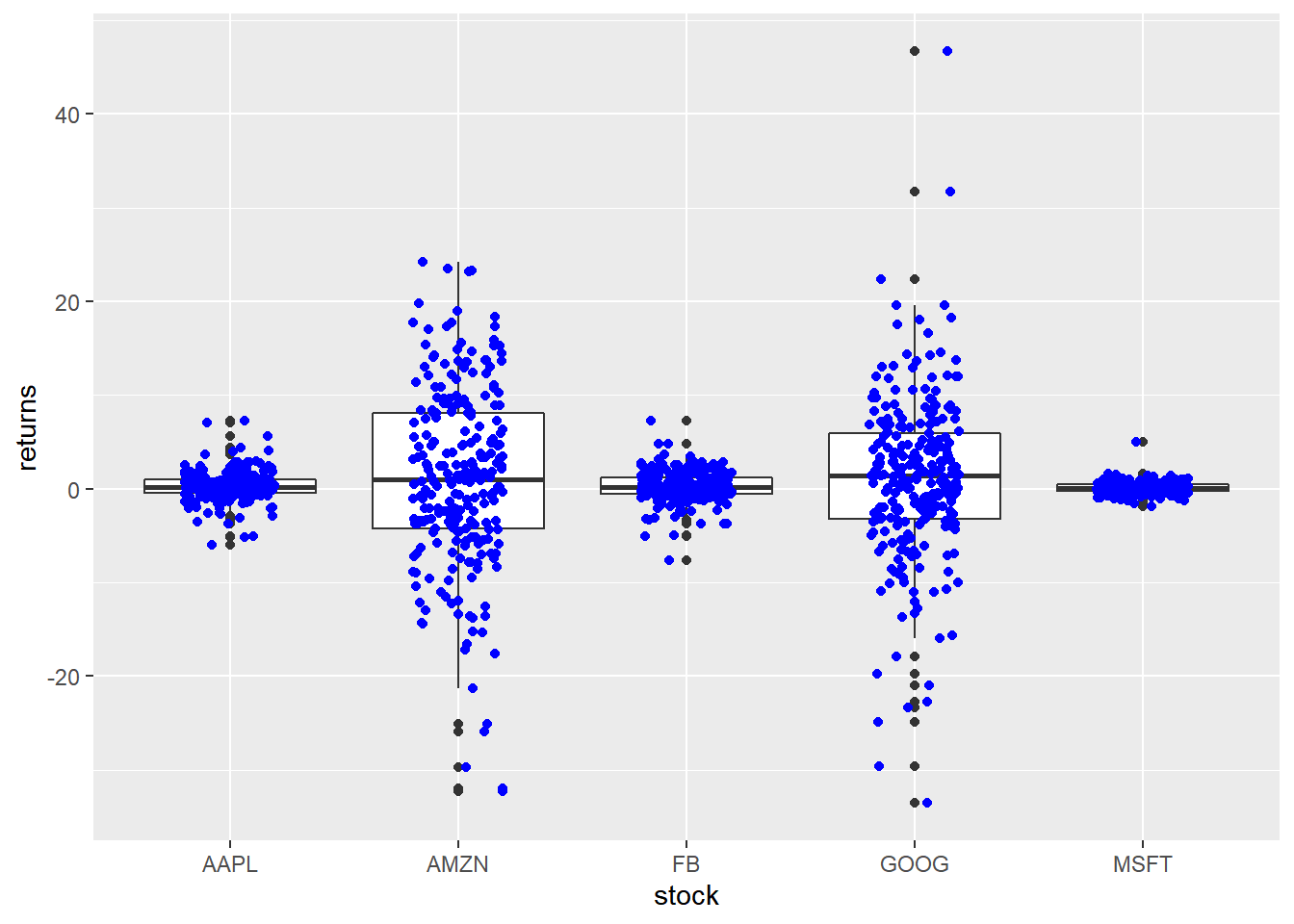## Outliers

To highlight extreme observations, we can modify the appearance of outliers
using the following:

• color
• shape
• size
• alpha

To modify the color of the outliers, use the `outlier.color` argument. The
color can be specified either using its name or the associated hex code.

```ggplot(tidy_returns) +
geom_boxplot(aes(x = stock, y = returns), outlier.color = 'red')```The shape of the outlier can be modified using the `outlier.shape` argument.
It can take values between `0` and `25`.

```ggplot(tidy_returns) +
geom_boxplot(aes(x = stock, y = returns), outlier.shape = 23) ```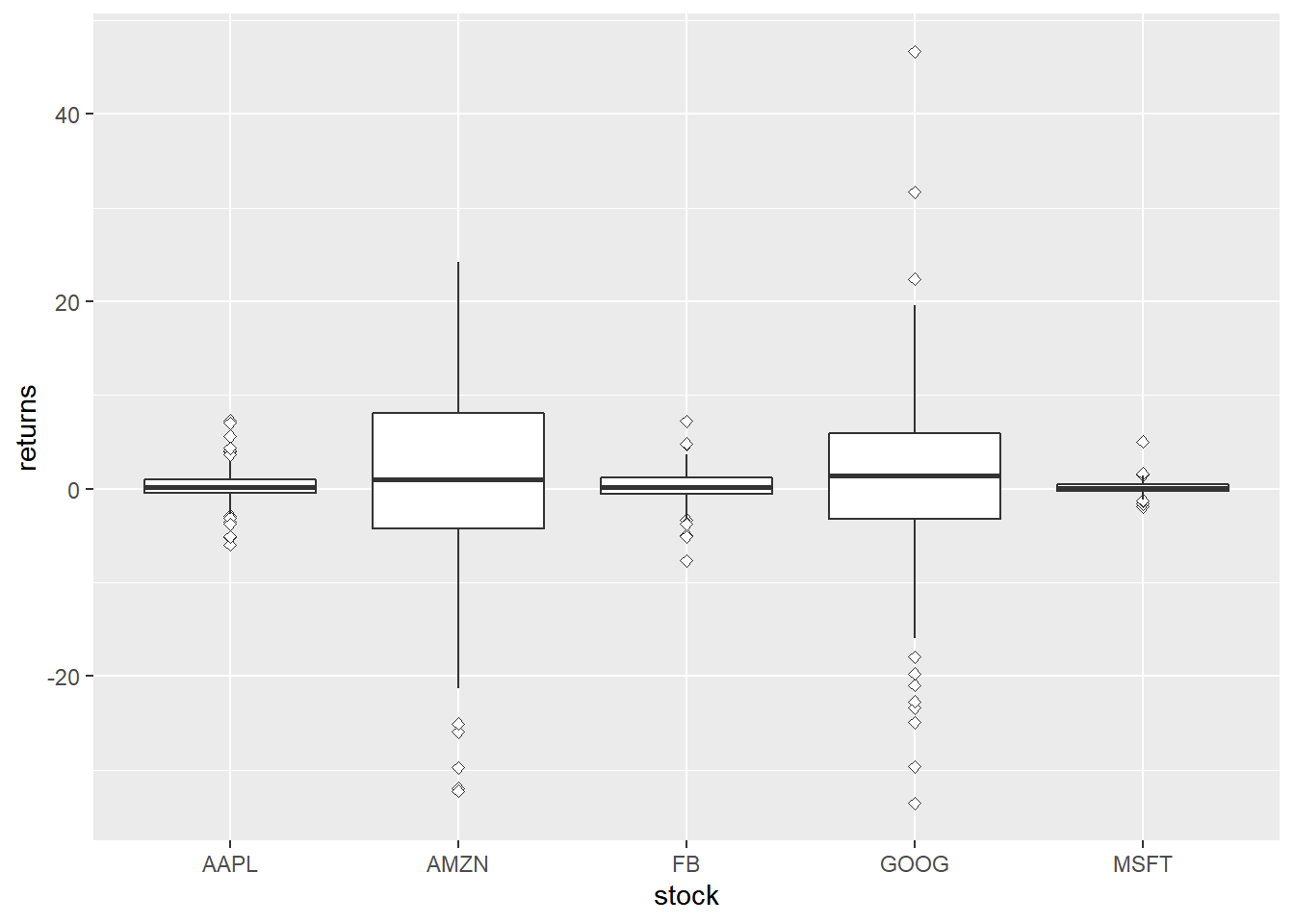The size of the outlier can be modified using the `outlier.size` argument. It
can take any value greater than `0`.

```ggplot(tidy_returns) +
geom_boxplot(aes(x = stock, y = returns), outlier.size = 3) ```You can play around with the transparency of the outlier using the
`outlier.alpha` argument. It can take values between `0` and `1`.

```ggplot(tidy_returns) +
geom_boxplot(aes(x = stock, y = returns), outlier.color = 'blue', outlier.alpha = 0.3) ```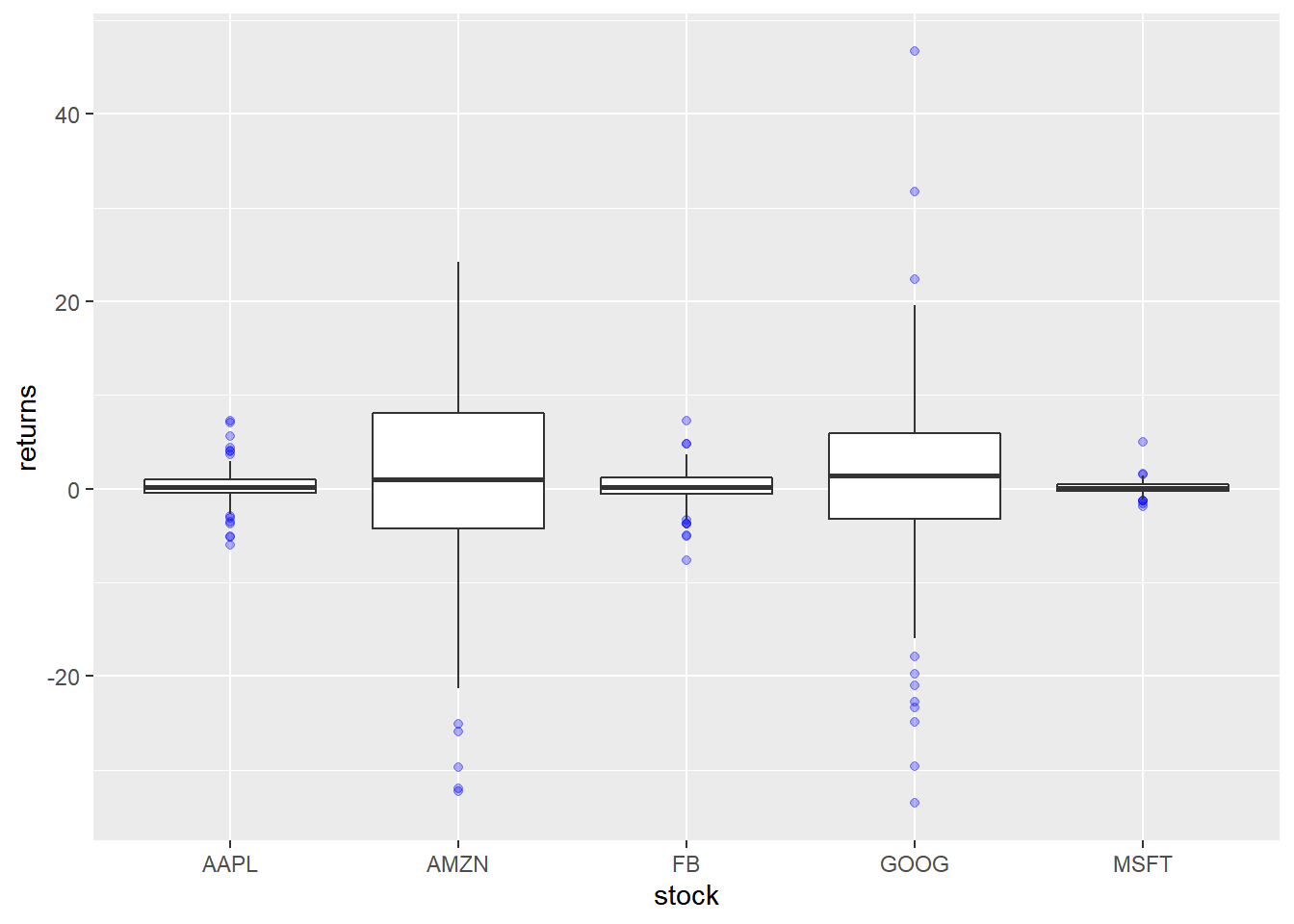## Box Aesthetics

The appearance of the box can be controlled using the following:

• color
• fill
• alpha
• line type
• line width

## Specify Values

The background color of the box can be modified using the `fill` argument. The
color can be specified either using its name or the associated hex code.

```ggplot(tidy_returns) +
geom_boxplot(aes(x = stock, y = returns), fill = c('blue', 'red', 'green', 'yellow', 'brown')) ```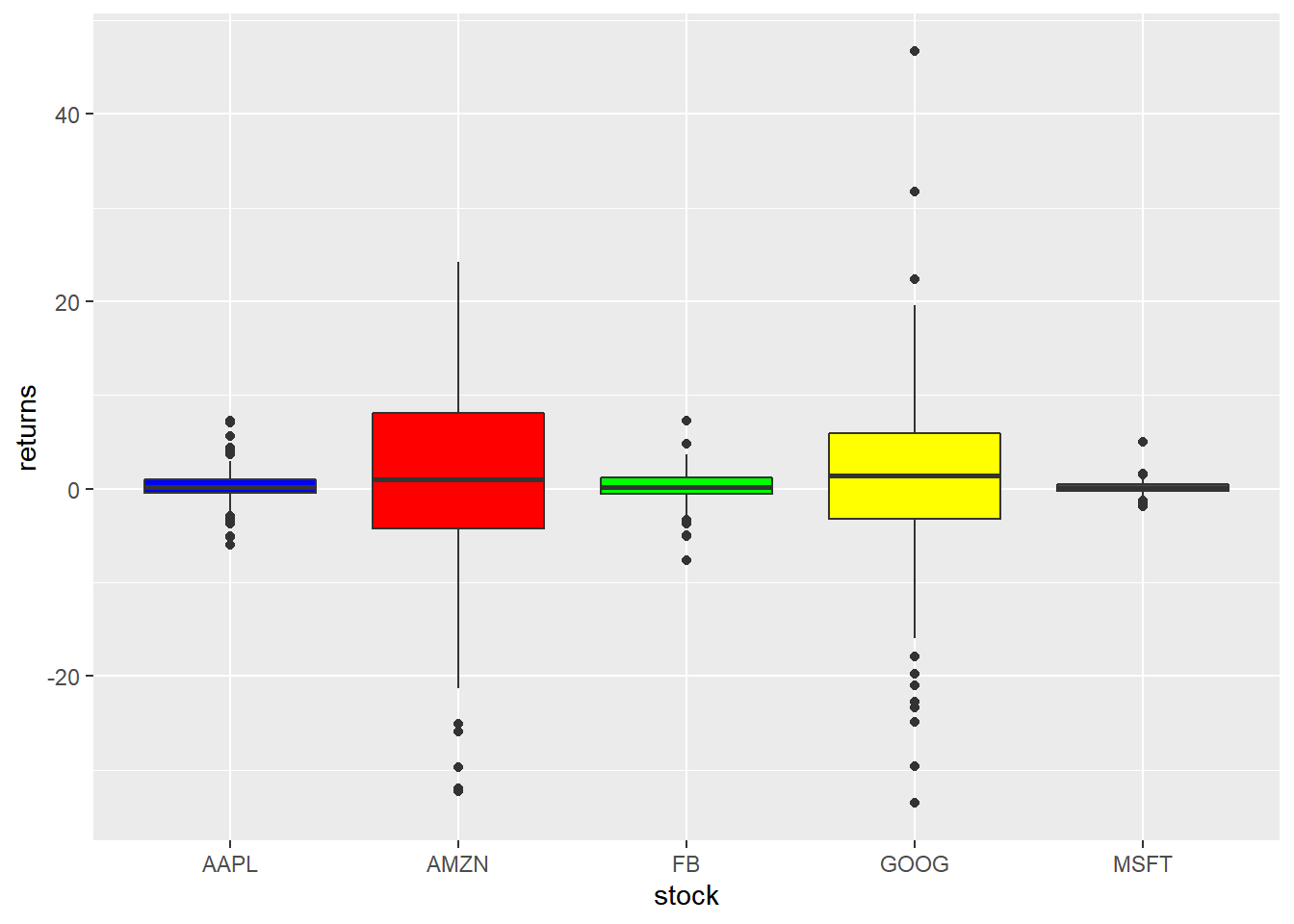To modify the transparency of the background color, use the `alpha` argument. It
can take any value between `0` and `1`.

```ggplot(tidy_returns) +
geom_boxplot(aes(x = stock, y = returns), fill = 'blue', alpha = 0.3) ```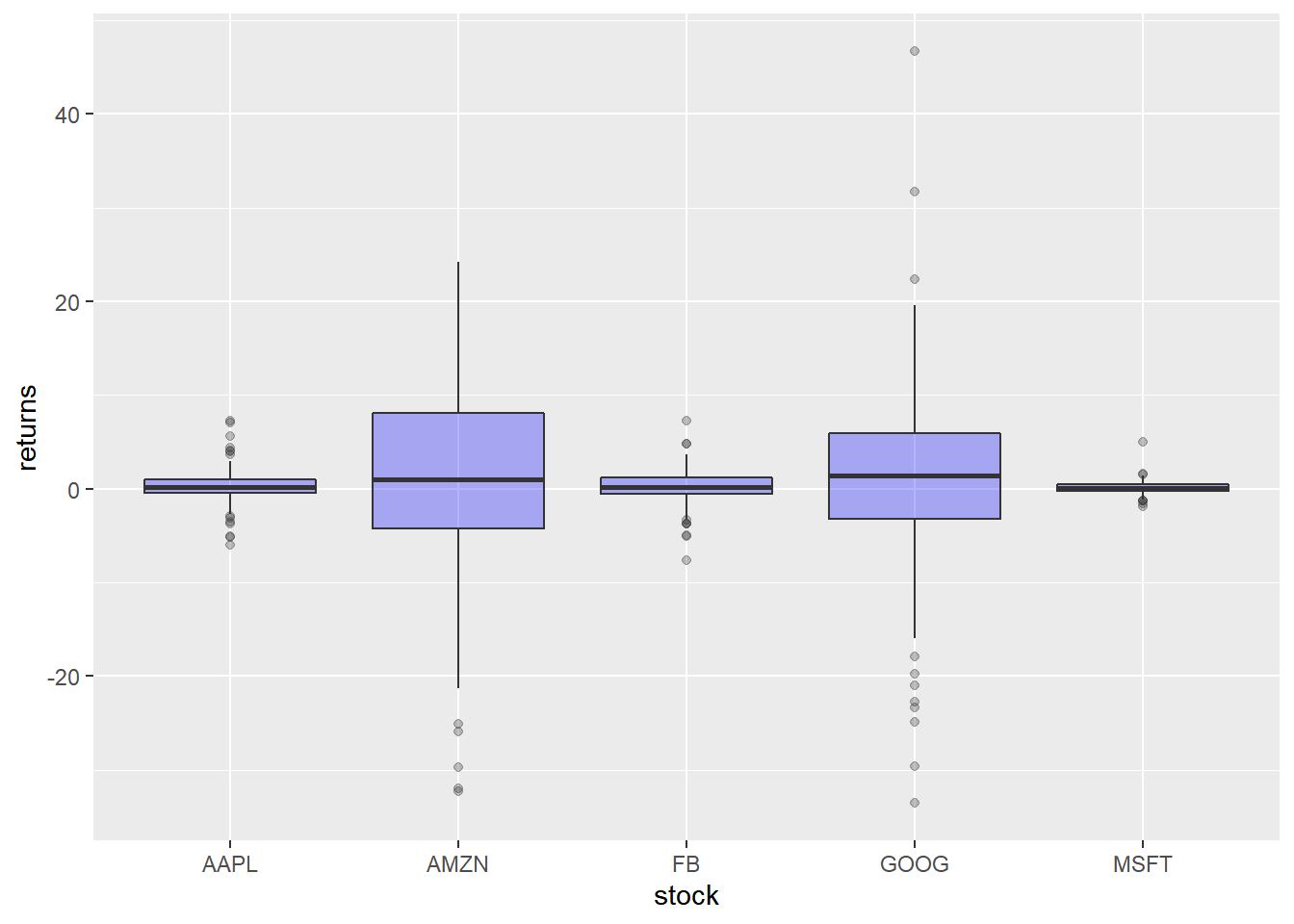The color of the border can be modified using the `color` argument. The
color can be specified either using its name or the associated hex code.

```ggplot(tidy_returns) +
geom_boxplot(aes(x = stock, y = returns), color = c('blue', 'red', 'green', 'yellow', 'brown')) ```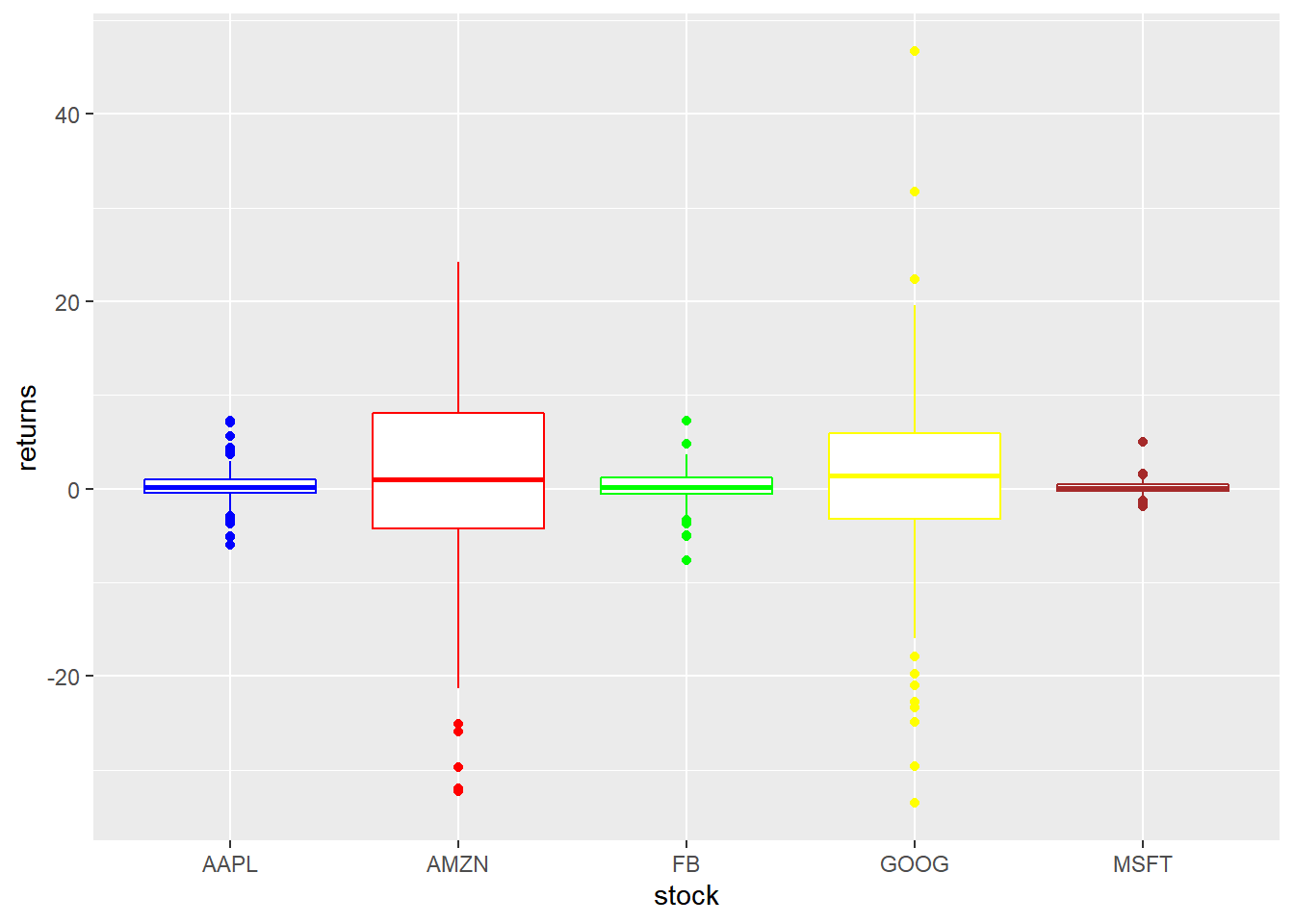The width of the border can be changed using the `size` argument. It can take
any value greater than `0`.

```ggplot(tidy_returns) +
geom_boxplot(aes(x = stock, y = returns), size = 1.5) ```To change the line type of the border, use the `linetype` argument. It can take
any value between `0` and `6`.

```ggplot(tidy_returns) +
geom_boxplot(aes(x = stock, y = returns), linetype = 2) ```## Map Variables

Instead of specifying values, we can map `fill` and `color` to variables as
well. In the below example, we map `fill` to the variable stock. It assigns
different colors to the different stocks.

```ggplot(tidy_returns) +
geom_boxplot(aes(x = stock, y = returns, fill = stock)) ```Let us map `color` to the variable stock. It will assign different colors
to the box borders.

```ggplot(tidy_returns) +
geom_boxplot(aes(x = stock, y = returns, color = stock)) ```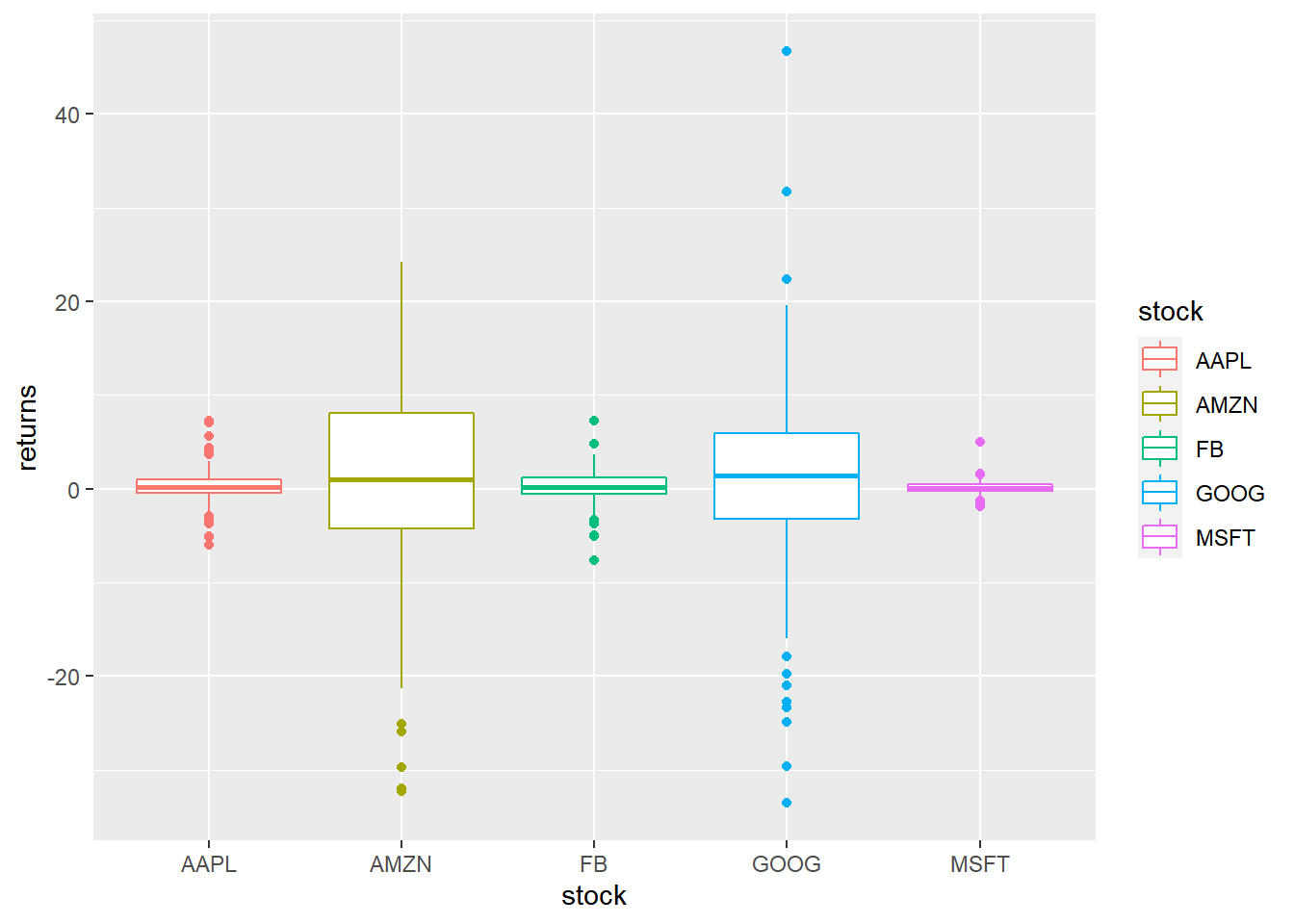### Summary

In this post, we learnt to:

• build box plots
• modify outlier color, shape, size etc.
• modify box color
• modify box line color, size and line type

### Up Next..

In the next post, we will learn to build histograms.